﻿ A Study about Solving the Nonlinear Spherical Seepage Model of Three-Area Composite Reservoir

### A Study about Solving the Nonlinear Spherical Seepage Model of Three-Area Composite Reservoir

Mei Luo, Shunchu li, Dongdong GuiOPEN ACCESSPEER-REVIEWED

## A Study about Solving the Nonlinear Spherical Seepage Model of Three-Area Composite Reservoir

Mei Luo1, Shunchu li1,, Dongdong Gui2

1School of Mathematics and Computer Engineering, Xihua University, Chengdu, China

2Beijing Dongrunke Petroleum Technology Co.,Ltd., Beijing, China

### Abstract

This paper studies the nonlinear spherical seepage model of three-area composite reservoir under three kinds of outer boundary conditions (infinite boundary, constant pressure boundary and closed boundary) which not considering well-bore storage and skin factor. On the basis of similar structure of solution for differential equation’s boundary value problem, this paper obtains the solution of the nonlinear spherical seepage model of three-area composite reservoir. The study not only benefits to understand the inherent laws of the solution, but also provides the convenience to compile corresponding test software.

• Luo, Mei, Shunchu li, and Dongdong Gui. "A Study about Solving the Nonlinear Spherical Seepage Model of Three-Area Composite Reservoir." American Journal of Applied Mathematics and Statistics 3.1 (2015): 17-22.
• Luo, M. , li, S. , & Gui, D. (2015). A Study about Solving the Nonlinear Spherical Seepage Model of Three-Area Composite Reservoir. American Journal of Applied Mathematics and Statistics, 3(1), 17-22.
• Luo, Mei, Shunchu li, and Dongdong Gui. "A Study about Solving the Nonlinear Spherical Seepage Model of Three-Area Composite Reservoir." American Journal of Applied Mathematics and Statistics 3, no. 1 (2015): 17-22.

 Import into BibTeX Import into EndNote Import into RefMan Import into RefWorks

### 1. Introduction

In recent years, mathematician have perfected the seepage theory. However, the problem of nonlinear seepage theory and application research are still at the primary stage. Error caused by the quadratic gradient effect is very big, which should not be ignored. while the traditional model of reservoir was established which not taking the quadratic gradient into consideration. This can cause larger error as a model of linear seepage. Therefore, solving the nonlinear seepage problem has important theoretical significance and practical value.

Ref 1 established the homogeneous reservoir seepage model, obtained the similar structure of solution based on the Theory of Similar Structure of Solution. Ref 2 put forward the similar structure of the solution to radial-spherical seepage problem considering quadratic gradient effect. Ref 3 discovered the similar constructive method of solutions of nonlinear seepage model for homogeneous reservoir. Ref 4 studied the similar constructive method for solving a nonlinearly spherical percolation model. Ref 5 discussed the similar constructive method of solution for the model of nonlinear spherical seepage in composite reservoir under the three kinds of outer boundary conditions (infinite, constant pressure and closed). Ref 6 studied how to solve the mathematical model of three-area composite reservoir.

Some of these researches studied the similar constructive method of solution for the model of nonlinear spherical seepage in composite reservoir, others discussed how to solve the mathematical model of three-area composite reservoir. Therefore, this paper establishes the nonlinear spherical seepage model of three-area composite reservoir under three kinds of outer boundary conditions (infinite boundary, constant pressure boundary and closed boundary), not considering well-bore storage and skin factor. Based on the theory of similar structure of solution, the solution of the nonlinear spherical seepage model of three-area composite reservoir can be obtained.

### 2. Establishing the Nonlinear Spherical Seepage Model of Three-Area Composite Reservoir

Here we study the model which not considering well-bore storage and skin factor. The main assumptions are as follows:

1) Single-phase micro compressible fluid obeys Darcy’s law;

2) Reservoir types is horizontal thickness, isotropic composite reservoir;

3) The well product is Isothermal flow instability;

4) At the interface of two seepage zone does not present the additional pressure drop;

5) Considering the effect of micro pressure gradient, neglecting the capillary single-phase horizontal flow without gravity effect;

6) Spherical seepage flow to a wall of the center reservoir.

The nonlinear spherical seepage equations of three-area composite reservoir which not considering well-bore storage and skin factor are as follows:

Inner area: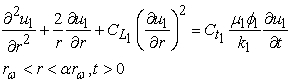(1)

Middle area: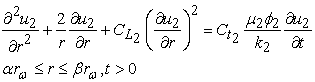(2)

Outer area: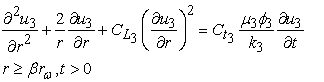(3)

Initial condition: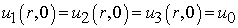(4)

Inner boundary condition: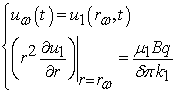(5)

Convergence condition: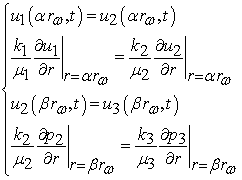(6)

Outer boundary condition:

1) When the outer boundary condition is infinite: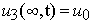(7)

2) When the outer boundary condition is constant pressure: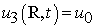(8)

3) When the outer boundary condition is closed: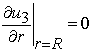(9)

### 3. Dimensionless the Seepage Mathematical Model

The dimensionless mathematical model of nonlinear spherical seepage of three-area composite reservoir is obtained: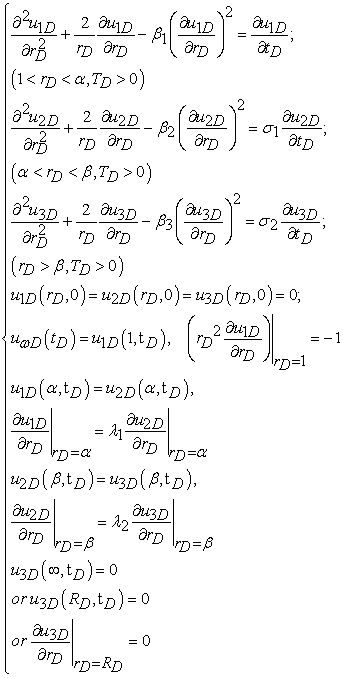(10)

Where dimensionless variables are introduced as follows: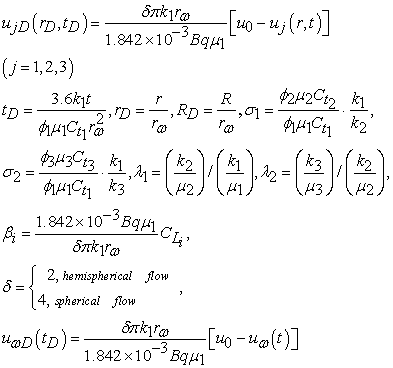### 4. Linearization of Nonlinear Seepage Mathematical Model

In order to make the seepage equation linearly, variable is replaced by the following: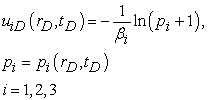(11)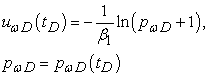(12)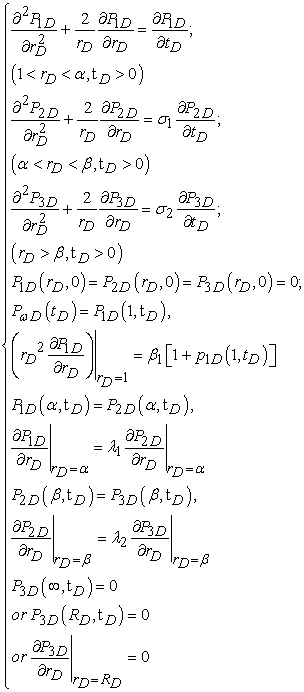(13)

### 5. Solution in Laplace Space and Similar Structure of the Solution

Take the Laplace transform on the dimensionless time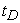, i.e.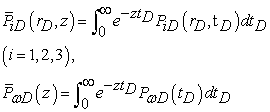(14)

Then the boundary value problem of the ODE in Laplace space with parameter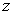(whereis Laplace space variable) is obtained: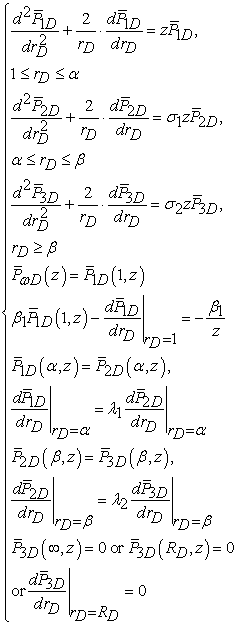(15)

### 6. Solving the Nonlinear Spherical Seepage Model of Three-Area Composite Reservoir

On the basis of the Similar Constructive Method, the Laplace space analytic solutions of each area of the boundary value problem (15) are obtained, respectively: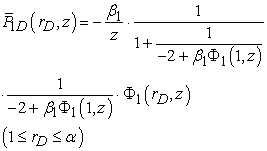(16)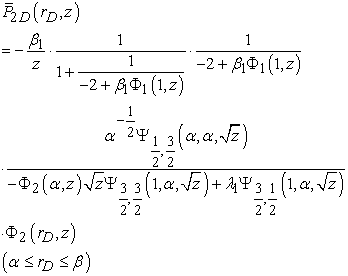(17)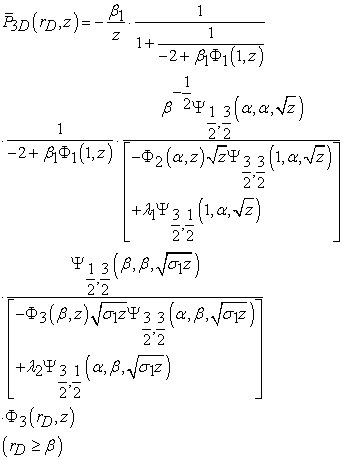(18)

Where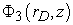are called the similar kernel function of outer area. Its expression is delimited as below:

1) When the outer boundary condition is infinite: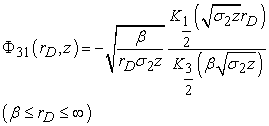(19)

2) when the outer boundary condition is constant: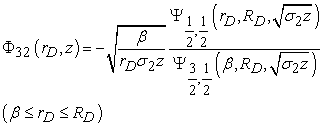(20)

3) when the outer boundary condition is closed: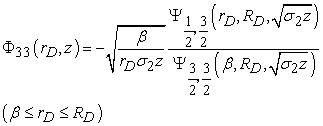(21)

Where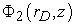are called the similar kernel function of middle area. Its expression is delimited as below: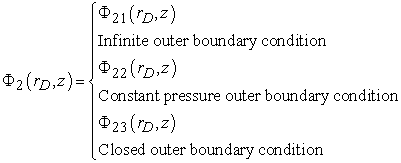1) When the outer boundary condition is infinite: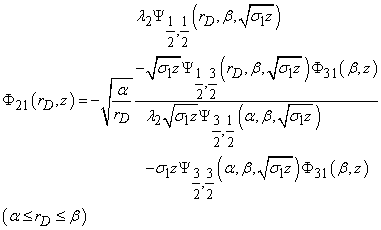(22)

2) when the outer boundary condition is constant: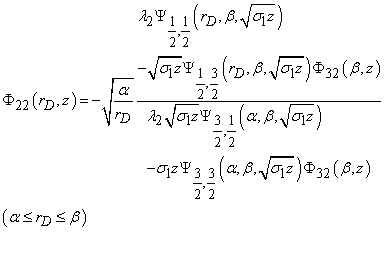(23)

3) when the outer boundary condition is closed: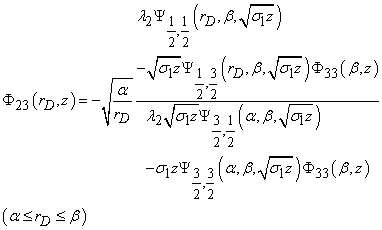(24)

Where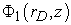are called the similar kernel function of inner area. Its expression is delimited as below:

1) when the outer boundary condition is infinite: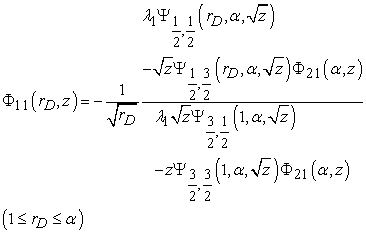(25)

2) when the outer boundary condition is constant: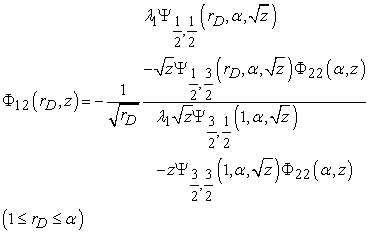(26)

3) when the outer boundary condition is closed: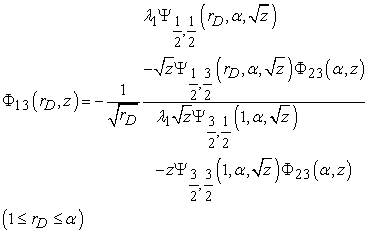(27)

Where,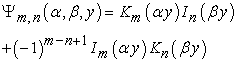(28)

And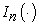,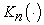are modified Bessel functions of order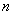.

Then let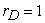, we can obtain the solution of dimensionless bottom-hole pressure in Laplace space: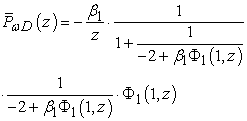(29)

By using Laplace inversion formula, we can obtain the solution of dimensionless pressure in the real space as follows: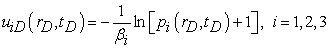Similarity, we can obtain the solution of dimensionless bottom-hole pressure in the real space as follows: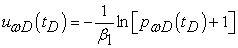### 7. Conclusions

Base on the theory of similar structure of the solution of the boundary value problem of differential equation, this paper gets the unified expression of reservoir pressure and bottom hole pressure solution of Laplace space under three different outer boundary conditions, and kernel function is only different in the expression for the different outer boundary conditions.

Many the nonlinear spherical seepage models of three-area composite reservoir can be adapted to the boundary value problem of three-area composite modified Bessel equation. So this paper can easily get the solution of the nonlinear spherical seepage model of three-area composite reservoir, after a simple algebra calculation.

In general, we can obtain the solution in the real space by using Laplace inversion formula, which is convenient to compile corresponding test software.

### Nomenclature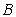—Formation volume factor (dimension);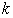—Permeability (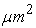);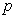—Reservoir pressure (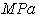);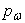—Well-bore pressure ();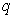—Production rate or injection rate (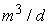);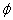—Porosity (dimensionless);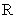—The outer boundary radius (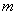);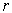—Well-bore radius ();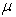—Viscosity (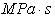);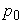—Initial reservoir pressure ();—Laplace space variable;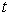—Time (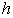);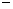—Laplace domain;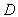—Dimensionless;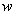—Well-bore parameter;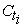—total compressibility (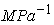);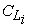—fluid compressibility (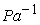);

### Acknowledgment

The research was supported by the Nature Science Key Projects of the Sichuan Education Bureau of China(Grant No.12ZA164).

### References

  S.C. Li, P.C.Zheng, Y.F.Zhang. Pure and Applied Mathematics 2006, 22 (4): 459-461.In article  L.Xu, S.C. Li, J.C.Wang. Journal of Chongqing Technology and Business University (Natural Science Edition), 2011, 28 (6): 585-589.In article  X.T.Bao, S.C. Li,X.X.Xiao, X.X.Dong. NATURAL GAS AND OIL, 2012, 30(65): 47-51.In article  Y.Wang, X.T.Bao, S.C. Li. TELKOMNIKA Indonesian Journal of Electrical Engineering, Vol. 11, No. 3, pp. 1393-1402, 2013.In article  F.J. Zhang, X.X.Xiao, S.C. Li, D.D. Gui, Q.Wang. Similar Constructive Method of Solution for the Model of Nonlinear Spherical Seepage in Composite Reservoir.In article  X.X. Dong, S.C. Li, D.D. Gui, F.J. Zhang.A Study on Solving the Seepage Flow Model of Three-Area Composite Reservoir.In article  Liu Shishi, Liu Shida. Special Function. Beijing: China Meteorological Press, 2002.In article  S.C. Li,B.G.Huang. Laplace Transformation and Bessel Function and the Theoretical Foundation of Well test Analytical, 2000: 87-123.In article  S.C. Li, X.Q.Wu. Journal of Xihua University (Natural Science Edition), 2013, 32 (4): 27-31.In article  J.C.Wang, S.C. Li, L.Xu. ournal of Guilin University of Technology, 2012, 32 (4): 624-627.In article